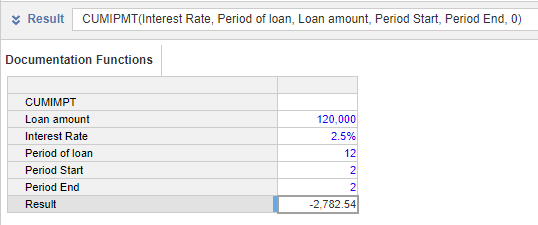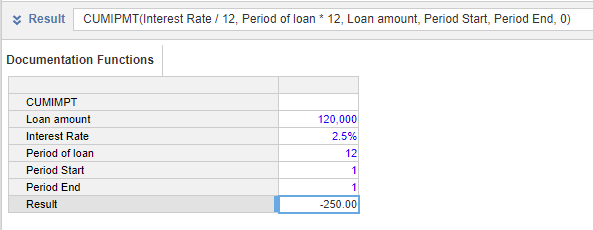1. Calculation functions
2. All Functions
3. Financial functions
4. CUMIPMTThis function calculates the cumulative interest paid on a loan during a specified period.

This function assumes equal payment amounts over the period of the loan.

The period passed in any argument in the function must be is consistent. The function takes up to six arguments: the first argument (i) requires the interest rate for the calculation period; the second (p), fourth(s), and fifth(e) arguments specify the periods used in the calculation. These periods must match, so to calculate the annual cumulative interest paid, use the annual interest rate, and the number of periods in years. Bear this in mind when using hard-coded values in the function.

This function is equivalent to the Excel function CUMIPMT.

Output

The function must be used in a line item with the Format: Number.

Syntax

CUMIPMT(i, p, v, s, e, [mode])
Argument Description Format Available Sources
i interest rate per period Number (percentage) Numeric hard-coded value, line item, or property
p the number of periods over which the loan is repaid Number Numeric hard-coded value, line item, or property
v the current loan balance Number Numeric hard-coded value, line item, or property
s the first payment period used in the calculation. Cumulative interest is calculated between this point and the end period. Number Numeric hard-coded value, line item, or property
e the calculation end period. Cumulative interest is calculated between the start period and this point. Number Numeric hard-coded value, line item, or property
mode (optional) when the payments are made. If the value is not provided, or the value is zero, the function assumes payments are made at the end of each period; a non-zero value means payments are made at the start of each period. Number

Numeric hard-coded value, line item, or property

Example

The formula to calculate the cumulative interest paid in the second year is:

CUMIPMT(Interest Rate, Period of loan, Loan amount, Period Start, Period End, 0)

where the line items use this data:

Line item Value
Interest Rate 2.5
Period of loan 12
Loan amount 120000
Period Start 2
Period End 2

Use the same figure for the start period and end period to find the interest for a single year.Using the same data, the formula to calculate the cumulative interest paid in the first month only is:

CUMIPMT(Interest Rate/12, Period of loan*12, Loan amount, Period Start, Period End, 0)

Because the data is in years, the period interest rate and the number of payments over the period of the loan must be recalculated. To do this:

• divide the Interest Rate by 12, and
• multiply the Period of loan by 12.